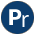#dif.pl -- The dif/2 constraint

dif(+Term1, +Term2) is semidet
Constraint that expresses that Term1 and Term2 never become identical (==/2). Fails if `Term1 == Term2`. Succeeds if Term1 can never become identical to Term2. In other cases the predicate succeeds after attaching constraints to the relevant parts of Term1 and Term2 that prevent the two terms to become identical.
dif_c_c(+X, +Y, !OrNode)[private]
Enforce `dif(X,Y)` that is related to the given OrNode. If X and Y are equal we reduce the OrNode. If they cannot unify we are done. Otherwise we extend the OrNode with new pairs and create/extend the vardif/2 terms for the left hand side of the unifier as well as the right hand if this is a variable.
dif_c_c_l(+Unifier, +OrNode)[private]
Extend OrNode with new elements from the unifier. Note that it is possible that a unification against the same variable appears as a result of how unifiable acts on sharing subterms. This is prevented by simplify_ornode/3.
- test 14 in `src/Tests/attvar/test_dif.pl`.
Extend the vardif constraints on X and Y with the OrNode.
simplify_ornode(+OrNode) is semidet[private]
Simplify the possible unifications left on the original dif/2 terms. There are two reasons for simplification. First of all, due to the way unifiable works we may end up with variables in the unifier that do not refer to the original terms, but to variables in subterms, e.g. `[V1 = f(a, V2), V2 = b]`. As a result of subsequent unifying variables, the unifier may end up having multiple entries for the same variable, possibly having different values, e.g., ```[X = a, X = b]```. As these can never be satified both we have prove of inequality.

Finally, we remove elements from the list that have become equal. If the OrNode is empty, the original terms are equal and thus we must fail.

To be done
- The simplification is complicated. Another approach would be to turn `[X1=Y1, X2=Y2, ...]` into `[X1,X2,...]` and `[Y1,Y2,...]` and call unifiable/3 on these lists to either end up with a contradiction (satisfied) or a new unifier. This is a stronger propagation. Seems not so easy though. Both pending constraints and reconnecting to the proper variables need attention.
attr_unify_hook(+VarDif, +Other)[private]
If two dif/2 variables are unified we must join the two vardif/2 terms. To do so, we filter the vardif terms for the ones involved in this unification. Those that are represent OrNodes that have a unification satisfied. For the rest we remove the unifications with self, append them and use this as new vardif term.

On unification with a value, we recursively call dif_c_c/3 using the existing OrNodes.

or_nodes_completed(+List) is semidet[private]
Unification may have made some of the or nodes internally inconsistent. This code checks for that and makes the or node succeed if this is the case.
verify_compounds(+Nodes, +Value)[private]
Unification to a concrete Value (no variable)
or_succeed(+OrNode) is det[private]
The dif/2 constraint related to OrNode is complete, i.e., some (sub)terms can definitely not become equal. Next, we can clean up the constraints. We do so by setting the OrNode to `-` and remove this dead OrNode from every vardif/2 attribute we can find.
or_one_fail(+OrNode) is semidet[private]
Some unification related to OrNode succeeded.